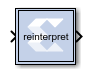# Vector Reinterpret - 2022.1 English

## Vitis Model Composer User Guide (UG1483)

Document ID
UG1483
Release Date
2022-05-26
Version
2022.1 English

The Vector Reinterpret block changes the vector input signal type without altering the binary representation. You can change the signal between signed and unsigned, and relocate the binary point.

Hardware notes: In hardware this block costs nothing.

Example: The input is 6 bits wide, signed with 2 fractional bits, and the output is forced to unsigned with 0 fractional bits. Then an input of -2.0 (1110.00 in binary 2's complement) becomes an output of 56 (111000 in binary).

## Description

Super Sample Rate (SSR): This configurable GUI parameter is primarily used to control processing of multiple data samples on every sample period. This block enables 1-D vector data support for the primary block operation.

The Vector Reinterpret block forces its output to a new type without any regard for retaining the numerical value represented by the input.The binary representation is passed through unchanged, so in hardware this block consumes no resources. The number of bits in the output will always be the same as the number of bits in the input.

The block allows for unsigned data to be reinterpreted as signed data, or, conversely, for signed data to be reinterpreted as unsigned. It also allows for the reinterpretation of the data's scaling, through the repositioning of the binary point within the data. The Xilinx Scale block provides an analogous capability.

An example of this block's use is as follows: if the input type is 6 bits wide and signed, with 2 fractional bits, and the output type is forced to be unsigned with 0 fractional bits, then an input of -2.0 (1110.00 in binary, two's complement) would be translated into an output of 56 (111000 in binary).

This block can be particularly useful in applications that combine it with the Xilinx Slice block or the Xilinx Concat block. To illustrate the block's use, consider the following scenario:

Given two signals, one carrying signed data, and the other carrying two unsigned bits (a `UFix_2_0`), you should design a system that concatenates the two bits from the second signal onto the tail (least significant bits) of the signed signal.

You can do so using two Vector Reinterpret blocks and one Vector Concat block. The first Vector Reinterpret block is used to force the signed input signal to be treated as an unsigned value with its binary point at zero. The result is then fed through the Vector Concat block along with the other signal's `UFix_2_0`. The Concat operation is then followed by a second Vector Reinterpret that forces the output of the Vector Concat block back into a signed interpretation with the binary point appropriately repositioned.

Though three blocks are required in this construction, the hardware implementation is realized as simply a bus concatenation, which has no cost in hardware.

## Block Parameters

Parameters specific to the block are as follows.

Force Arithmetic Type
When checked, the Output Arithmetic Type parameter can be set and the output type is forced to the arithmetic type chosen according to the setting of the Output Arithmetic Type parameter. When unchecked, the arithmetic type of the output is unchanged from the arithmetic type of the input.
Output Arithmetic Type
The arithmetic type (unsigned or signed, 2's complement, Floating-point) to which the output is to be forced.
Force Binary Point
When checked, the Output Binary Point parameter can be set and the binary point position of the output is forced to the position supplied in the Output Binary Point parameter. When unchecked, the arithmetic type of the output is unchanged from the arithmetic type of the input.
Output Binary Point
The position to which the output's binary point is to be forced. The supplied value must be an integer between zero and the number of bits in the input (inclusive).